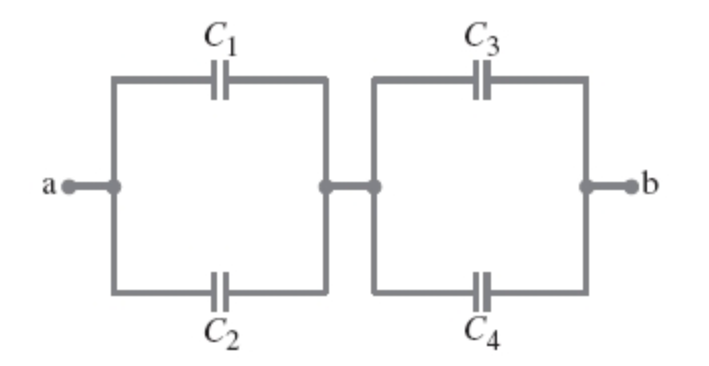# Problem: Determine the equivalent capacitance between points a and b for the combination of capacitors shown in Express your answer in terms of the variables C1, C2, C3, and C4.

###### FREE Expert Solution

For capacitors in parallel, the equivalent capacitance is:

$\overline{){{\mathbf{C}}}_{\mathbf{e}\mathbf{q}}{\mathbf{=}}{{\mathbf{C}}}_{{\mathbf{1}}}{\mathbf{+}}{{\mathbf{C}}}_{{\mathbf{2}}}{\mathbf{+}}{\mathbf{.}}{\mathbf{.}}{\mathbf{.}}{\mathbf{+}}{{\mathbf{C}}}_{{\mathbf{n}}}}$

83% (304 ratings)###### Problem Details

Determine the equivalent capacitance between points a and b for the combination of capacitors shown inExpress your answer in terms of the variables C1, C2, C3, and C4.